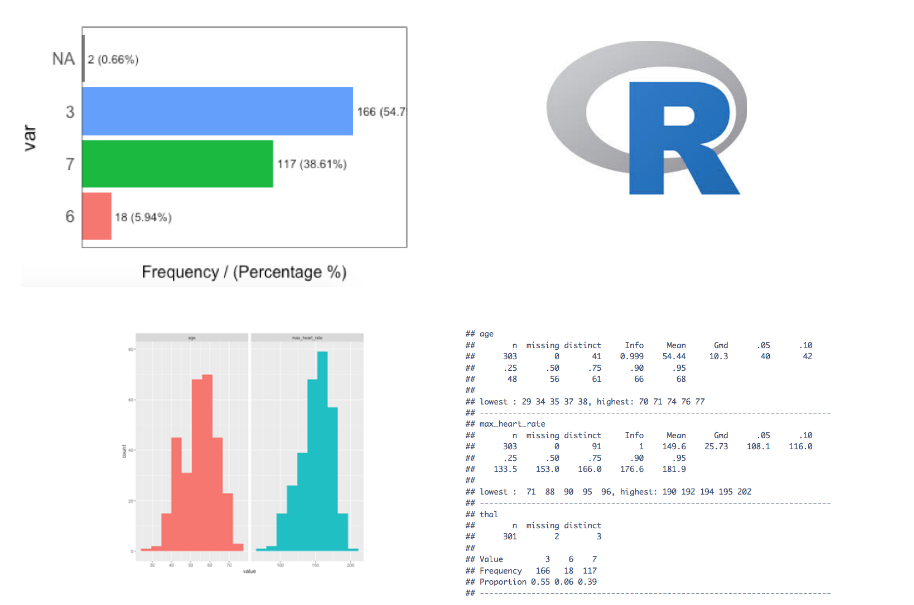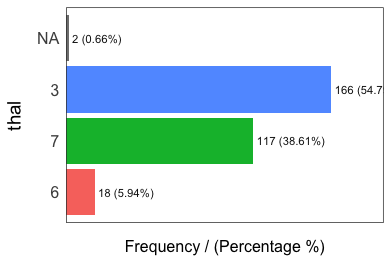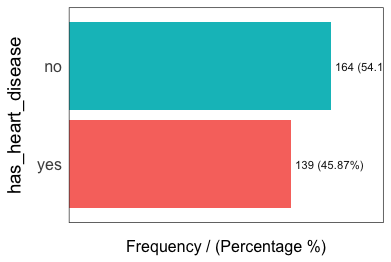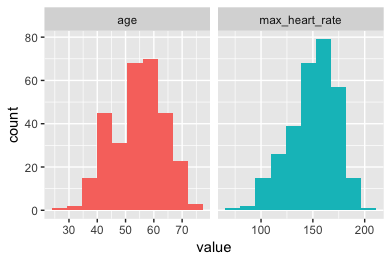# Exploratory Data Analysis in R (Introduction)

### Exploratory data analysis (EDA) is the very first step in a data project. We will create a code template to achieve this with one function.

· Big Data Zone · Tutorial
Save
8.76K Views## Introduction

EDA consists of univariate (1-variable) and bivariate (2-variables) analysis.
In this post, we will review some functions that lead us to the analysis of the first case.

• Step 1 - First approach to data.
• Step 2 - Analyzing categorical variables.
• Step 3 - Analyzing numerical variables.
• Step 4 - Analyzing numerical and categorical at the same time.

Covering some key points in a basic EDA:

• Data types.
• Outliers.
• Missing values.
• Distributions (numerically and graphically) for both, numerical and categorical variables.

## Type of Analysis Results

There are two type of result: informative or operative.

• Informative: For example plots, or any long variable summary. We cannot filter data from it, but it gives us a lot of information at once. Most be used at the EDA stage.
• Operative: The results can be used to take an action directly on the data workflow (for example, selecting any variables whose percentage of missing values are below 20%). Most used in the Data Preparation stage.

## Getting Set Up

Uncoment in case you don't have any of these libraries:

``````# install.packages("tidyverse")
# install.packages("funModeling")
# install.packages("Hmisc")``````

A newer version of `funModeling` has been released on Ago-1, please update!

``````library(funModeling)
library(tidyverse)
library(Hmisc)``````

### tl;dr (Code)

Run all the functions in this post in one-shot with the following function:

``````basic_eda <- function(data)
{
glimpse(data)
df_status(data)
freq(data)
profiling_num(data)
plot_num(data)
describe(data)
}``````

Replace `data` with your data, and that's it!

`basic_eda(my_amazing_data)`

Creating the data for this example:

Using the `heart_disease` data (from `funModeling` package). We will take only 4 variables for legibility.

``data=heart_disease %>% select(age, max_heart_rate, thal, has_heart_disease)``

## Step 1: The First Approach to the Data

Number of observations (rows) and variables, and a `head` of the first cases.

``glimpse(data)``
``````## Observations: 303
## Variables: 4
## \$ age               <int> 63, 67, 67, 37, 41, 56, 62, 57, 63, 53, 57, ...
## \$ max_heart_rate    <int> 150, 108, 129, 187, 172, 178, 160, 163, 147,...
## \$ thal              <fct> 6, 3, 7, 3, 3, 3, 3, 3, 7, 7, 6, 3, 6, 7, 7,...
## \$ has_heart_disease <fct> no, yes, yes, no, no, no, yes, no, yes, yes,...``````

Getting the metrics about data types, zeros, infinite numbers, and missing values:

``df_status(data)``
``````##            variable q_zeros p_zeros q_na p_na q_inf p_inf    type unique
## 1               age       0       0    0 0.00     0     0 integer     41
## 2    max_heart_rate       0       0    0 0.00     0     0 integer     91
## 3              thal       0       0    2 0.66     0     0  factor      3
## 4 has_heart_disease       0       0    0 0.00     0     0  factor      2``````

`df_status` returns a table, so it is easy to keep with variables that match certain conditions like:

• Having at least 80% of non-NA values (`p_na < 20`).
• Having less than 50 unique values (`unique <= 50`).

TIPS:

• Are all the variables in the correct data type?
• Variables with lots of zeros or `NA`s?
• Any high cardinality variable?

## Step 2: Analyzing Categorical Variables

`freq` function runs for all factor or character variables automatically:

``freq(data)````````##   thal frequency percentage cumulative_perc
## 1    3       166      54.79              55
## 2    7       117      38.61              93
## 3    6        18       5.94              99
## 4 <NA>         2       0.66             100````````````##   has_heart_disease frequency percentage cumulative_perc
## 1                no       164         54              54
## 2               yes       139         46             100``````
``##  "Variables processed: thal, has_heart_disease"``

TIPS:

• If `freq` receives one variable —`freq(data\$variable)`— it retruns a table. This is useful for treating high cardinality variables (like zip code).
• Export the plots to jpeg format in the current directory: `freq(data, path_out = ".")`
• Do all the categories make sense?
• Are there lots of missing values?
• Always check the absolute and relative values

## Step 3: Analyzing Numerical Variables

We will see: `plot_num` and `profiling_num`. Both run automatically for all numerical/integer variables:

### Graphically

``plot_num(data)``Export the plot to jpeg: `plot_num(data, path_out = ".")`

TIPS:

• Try to identify high-unbalanced variables.
• Visually check any variable with outliers.

### Quantitatively

`profiling_num` runs for all numerical/integer variables automatically:

``data_prof=profiling_num(data)``
``````##         variable mean std_dev variation_coef p_01 p_05 p_25 p_50 p_75 p_95
## 1            age   54       9           0.17   35   40   48   56   61   68
## 2 max_heart_rate  150      23           0.15   95  108  134  153  166  182
##   p_99 skewness kurtosis iqr        range_98     range_80
## 1   71    -0.21      2.5  13        [35, 71]     [42, 66]
## 2  192    -0.53      2.9  32 [95.02, 191.96] [116, 176.6]``````

TIPS:

• Try to describe each variable based on its distribution (also useful for reporting).
• Pay attention to variables with high standard deviation.
• Select the metrics that you are most familiar with: `data_prof %>% select(variable, variation_coef, range_98)`: A high value in `variation_coef` may indictate outliers. `range_98` indicates where most of the values are.

## Step 4: Analyzing Numerical and Categorical at the Same Time

`describe` from Hmisc package.

``````library(Hmisc)
describe(data)``````
``````## data
##
##  4  Variables      303  Observations
## ---------------------------------------------------------------------------
## age
##        n  missing distinct     Info     Mean      Gmd      .05      .10
##      303        0       41    0.999    54.44     10.3       40       42
##      .25      .50      .75      .90      .95
##       48       56       61       66       68
##
## lowest : 29 34 35 37 38, highest: 70 71 74 76 77
## ---------------------------------------------------------------------------
## max_heart_rate
##        n  missing distinct     Info     Mean      Gmd      .05      .10
##      303        0       91        1    149.6    25.73    108.1    116.0
##      .25      .50      .75      .90      .95
##    133.5    153.0    166.0    176.6    181.9
##
## lowest :  71  88  90  95  96, highest: 190 192 194 195 202
## ---------------------------------------------------------------------------
## thal
##        n  missing distinct
##      301        2        3
##
## Value         3    6    7
## Frequency   166   18  117
## Proportion 0.55 0.06 0.39
## ---------------------------------------------------------------------------
## has_heart_disease
##        n  missing distinct
##      303        0        2
##
## Value        no  yes
## Frequency   164  139
## Proportion 0.54 0.46
## ---------------------------------------------------------------------------``````

Really useful to get a quick picture of all the variables. But is not as operative as `freq` and `profiling_num` when we want to use its results to change our data workflow.

TIPS:

• Check min and max values (outliers).
• Check Distributions (same as before).

That's all for now!

----

Pablo Casas.

---

Other posts you might like:

Topics:
r, machine learning, data science, exploratory data analysis

Published at DZone with permission of Pablo Casas, DZone MVB.

Opinions expressed by DZone contributors are their own.